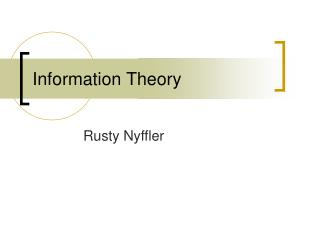DownloadDownload PresentationInformation Theory

# Information Theory

Download Presentation## Information Theory

- - - - - - - - - - - - - - - - - - - - - - - - - - - E N D - - - - - - - - - - - - - - - - - - - - - - - - - - -
##### Presentation Transcript

1. Information Theory Rusty Nyffler

2. Introduction • Entropy • Example of Use • Perfect Cryptosystems • Common Entropy

3. Entropy • Measure of uncertainty • Entropy of a fair coin toss = 1

4. Why is entropy important? • Measures the likelihood of the eavesdropper knowing something about the key, given the ciphertext • Greater entropy usually means greater security • Entropy of a cryptosystem (K = number of possible keys (if all keys are equally likely)

5. Example • 3 possible plaintexts: a(.5), b(.3), c(.2) • Two keys k1and k2, equally likely (.5) • Possible ciphertexts U,V,W • If someone obtains sent ciphertext, then information is known about the plaintext. • If U was received, then a was the text • If V was received, then b or c was the text

6. Example Continued • Moreover, • p(b|V) = .6 • p(c|V) = .4 • p(b|W) = .6 • p(c|W) = .4 • Which means it’s more likely that b is the plaintext when V or W is caught • Entropy shows that the eavesdropper knows more about the plaintext when the ciphertext is intercepted

7. Perfect Cryptosystems • In a perfect cryptosystem (i.e. unbreakable), the ciphertext should not give any more information about the plaintext or the key • H(P) = H(P|C) • H(K) = H(K|C)

8. Common Entropy • The English language • Somewhere between 1.42 and .72 • English is around 75% redundant • In many algorithms, the longer the key, the more entropy the system has • Vigenere

9. Common Entropy • RSA • Entropy = 0 • All the info you need is in n, e, and c … except it’ll take a while to factor n

10. References • MacKay, David J.C. Information Theory, Inference, and Learning Algorithms. 18 April 2003. http://www.inference.phy.cam.ac.uk/itprnn/book.l.pdf • North Carolina State University. Cryptography FAQ. 21 March 2003. http://isc.faqs.org/faqs/cryptography-faq/part01/ • Raynal, Frederick. Weak Algorithms. 2002. http://www.owasp.org/asac/cryptographic/algorithms.shtml • Trappe, Wade and Lawrence C. Washington. Introduction to Cryptography with Coding Theory. Upper Saddle River, New Jersey: Prentice-Hall, Inc., 2002.